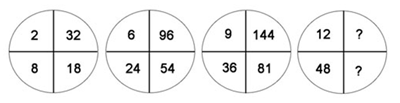# Find the Missing Number# Complete The Star

Find the missing numbers: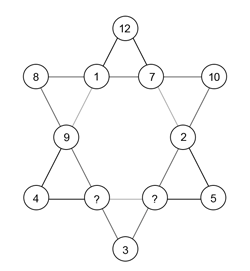# Filling Of Mugs

Remove 6 liters of oil from a large oil container using only two mugs of 9 liter and 4 liter respectively.

# Secret Locker Code

A man forgot the secret code to open his locker. But he remembers the following clues:

• Fifth number plus the third number equals fourteen.
• First number is one less than twice the second number.
• Fourth number is one more than the second number.
• Second number plus the third number equals ten.
• Sum of all five numbers is 30.

Can you find out the secret code to help him out?

# Missing Numbers

Find the missing value: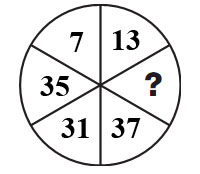# Number In Circles

Put the numbers from 1-9 in the circles in such a manner that all the sides of the triangle add up to 17.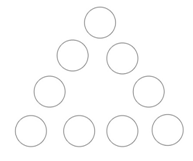# Number Games

Fill in the boxes with numbers from 1-10. One number can be used only once.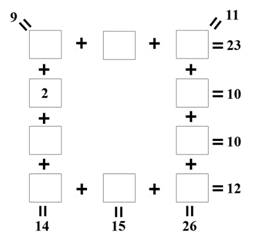# Smallest 4 Digit Number

Find the smallest 4 digit number meeting the following criteria:

1. First digit is 1/3 of the second digit.
2. Third digit is the sum of the first and second digit.
3. The last digit is thrice the second digit.

Tags: ,

# What Next?

Fill in the next line:

9 7 5 9 4 9
6 3 4 5 3 6
1 8 2 0 1 8

_______